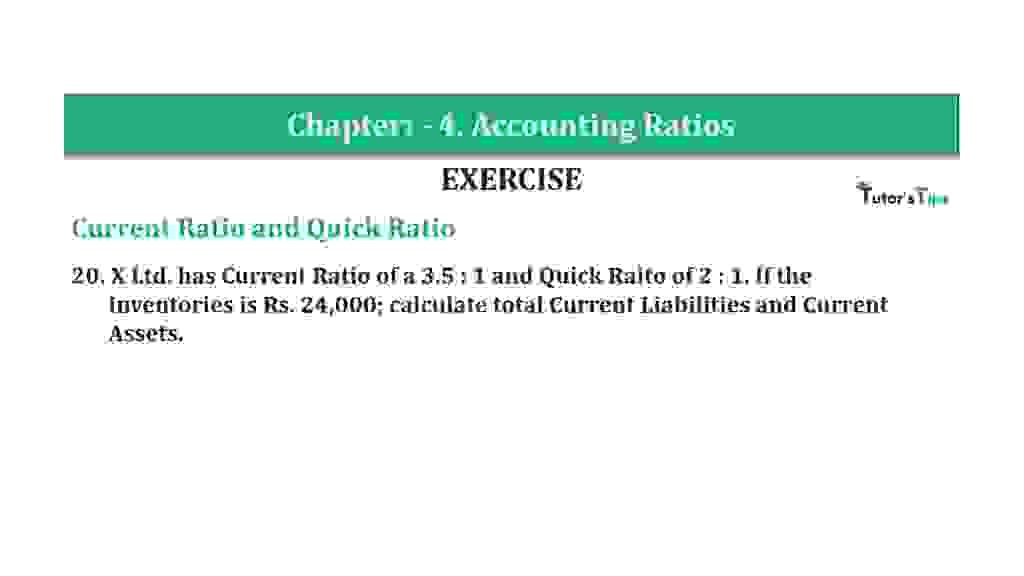# Question 20 Chapter 4 of +2-B – T.S. Grewal 12 ClassQuestion 20 Chapter 4 of +2-B

Current Ratio and Quick Ratio

20. X Ltd. has Current Ratio of a 3.5: 1 and Quick Raito of 2: 1. If the
Inventories are Rs. 24,000; calculate total Current Liabilities and Current
Assets.

### The solution of Question 20 Chapter 4 of +2-B: –

 Current Ratio = Current Assets = 3.5 Current Liabilities 1

 Quick Ratio = Quick Assets = 2 Current Liabilities 1

 Let Current Liabilities = x Current Assets = 3.5x Quick Assets = 2x Inventories = Current Assets – Quick Assets Rs. 24,000 = 3.5x – 2x Rs. 24,000 = 1.5x x = Rs. 16,000 Current Liabilities = X = Rs. 16,000

 Current Assets = 3.5 x Rs.16,000 = Rs. 56,000

Balance Sheet: Meaning, Format & Examples

Thanks, Please Like and share with your friends

Comment if you have any question.

Also, Check out the solved question of previous Chapters: –

### T.S. Grewal’s Double Entry Book Keeping (Vol. II: Accounting for Companies)T.S. Grewal’s Analysis of Financial Statements